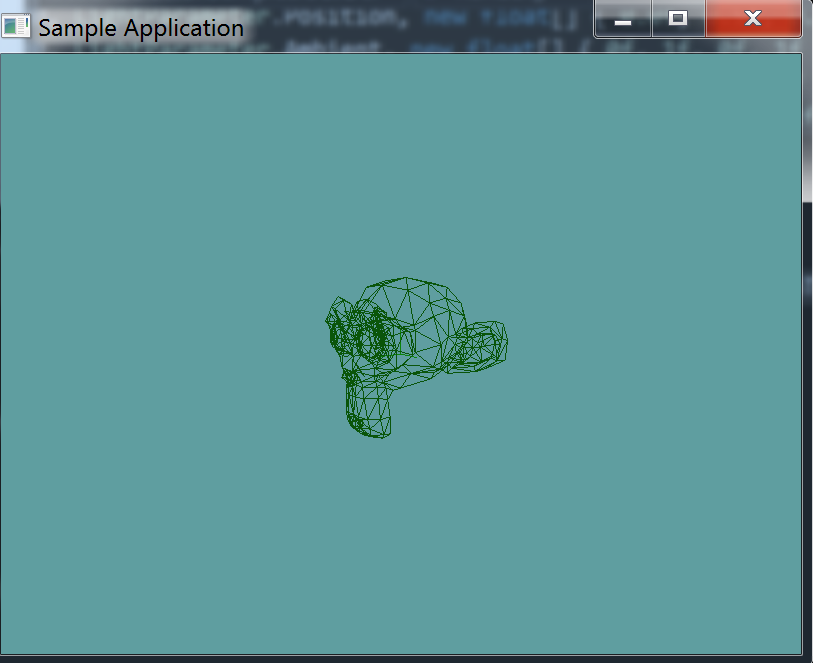It's time to start implementing some broad phase collision. The first thing we're going to do is add a list of triangles to our 3D model. This is going to be done at load time, as the models vertex array is filled in, we will also fill in a list of triangles.

We're also going to add a "DebugRender" function, that will loop trough all the triangles and render them. Because this is going to render each triangle indevidually, it's going to be much, much slower than the regular render function.

## Constructing Triangles

First thing is first, add a triangle array as a member variable to the ObjLoader class. I called mine collisionMesh

protected Triangle[] collisionMesh = null;


Now, in the actual constructor, we have to fill this array in. The constructor has a local array, called vertexData, it's a bunch of floats. Once that array is filled in, it contains sequential triangles.

That is, the first triangle of the model is made out of the first 9 elements of the array, like so:

• new Triangle(
• new Point(vertexData, vertexData, vertexData),
• new Point(vertexData, vertexData, vertexData),
• new Point(vertexData, vertexData, vertexData),
• )

That means that you have as many triangles as the size of that array, divided by 9. In the case of the suzanne model, that's 968. You can allocate the array like so:

collisionMesh = new Triangle[vertexData.Count / 9];


Figure out a way to loop trough vertex data in a way that fills the collisionMesh array with proper data. Once you think you have that done, implement the debug renderer from below and run the unit test.

## Debug Render

First, let's add a debug getter to check how many debug triangles we have

public int NumCollisionTriangles {
get {
return collisionMesh.Length;
}
}


Next, a "debug render" method, this method will simply draw out all triangles:

 public void DebugRender() {
foreach(Triangle trianlge in collisionMesh) {
trianlge.Render();
}
}


### Unit Test

You can Download the samples for this chapter to see if your result looks like the unit test.

You should already have suzanne in your project from earlyer in this chapter. This section just added triangles to the OBJLoader code. The constructor only errors out if the triangle count is off. Otherwise the rest of the test is visual only. You should see susane in the testusing OpenTK.Graphics.OpenGL;
using Math_Implementation;
using CollisionDetectionSelector.Primitives;
using CollisionDetectionSelector;

namespace CollisionDetectionSelector.Samples {
class OBJDebugTriangles : Application {

public override void Intialize(int width, int height) {
GL.Enable(EnableCap.DepthTest);
GL.Enable(EnableCap.CullFace);
GL.Enable(EnableCap.Lighting);
GL.Enable(EnableCap.Light0);

GL.PolygonMode(MaterialFace.FrontAndBack, PolygonMode.Line);

GL.Light(LightName.Light0, LightParameter.Position, new float[] { 0.0f, 0.5f, 0.5f, 0.0f });
GL.Light(LightName.Light0, LightParameter.Ambient, new float[] { 0f, 1f, 0f, 1f });
GL.Light(LightName.Light0, LightParameter.Diffuse, new float[] { 0f, 1f, 0f, 1f });
GL.Light(LightName.Light0, LightParameter.Specular, new float[] { 1f, 1f, 1f, 1f });

if (obj.NumCollisionTriangles != 968) {
LogError("Suzanne triangle count expected at 968, got: " + obj.NumCollisionTriangles);
}
}

public override void Render() {
base.Render();
DrawOrigin();

GL.PushMatrix();
GL.Scale(3.0f, 3.0f, 3.0f);
obj.DebugRender();
GL.PopMatrix();
}
}
}